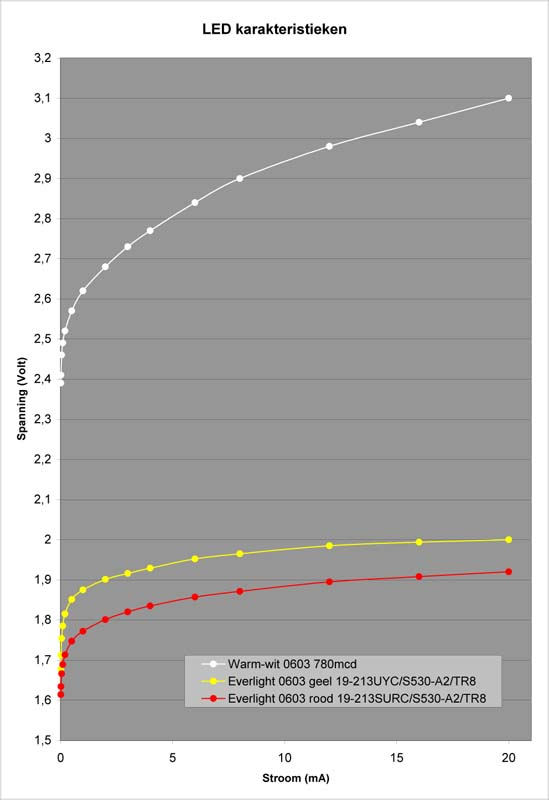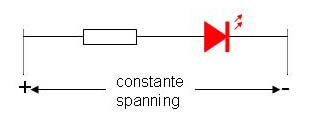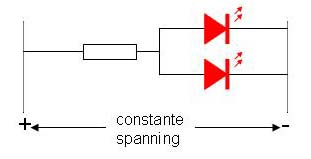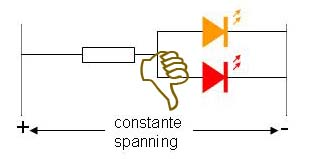# LEDs, a little refresh

A LED is a diode. A special one, because it produces light. A diode passes current in only one direction, a LED too. In forward direction a diode has a maximum current, if you exceed the maximum, it breaks dowm, a LED too. In reverse direction the diode blocks a maximum voltage, if you exceed the maximum, it breaks dowm, a LED too.

To pass current in forward direction, the diode needs a certain voltage. It is often said that the voltage across a diode is constant, with a silicon diode approximately 0.7 volts. That's about right, although not completely. For those of you who payed some attention during mathematics: The forward voltage of a diode is a logarithmic function of the current that passes through the diode. When the current approaches the maximum the diode can handle (say in the range between 1% and 100% thereof) the voltage varies hardly and is for the silicon diode approximately 0.7 volts

So, a LED is a diode. It just adds some light. We will not go into the exact physical background how that light is produced, otherwise you also should have payed attention during physics. Fact however is that the forward voltage of the LED depends on the wavelength it produces. A red LED (long wavelength) has a lower forward voltage than a yellow LED and that has a lower forward voltage than an ultra violet LED. The shorter the wavelength (and thus the higher the energy of the photons produced by the LED) the higher the forward voltage will be.

Hold on there, ultraviolet LEDs, who actually uses them? well, actually we do quite often, namely as white LEDs. A white LED actually is an ultra violet LED with a phosphor layer over it. The same kind of stuff as found on the inside of a fluorescent tube. A LED can produce only one wavelength of light and white light is a mixture of many different colors. The phosphor layer converts the UV radiation into visible light. By changing the mixture of the phosphor, the ratio of wavelengths in the light can be manipulated and thus the color temperature of the LED (cool white, warm white, etc).

# Voltage and current

So LEDs of different colors have different characteristics. This characteristic can be measured. The following image shows an example of a red, yellow and white LED at room temperature (click for a larger version). Note: this does not mean that all LEDs have this characteristic, it may very well vary by brand and type!Because the voltage-current characteristic (in forward direction) of a LED is "knee-shaped", it is not very wise to drive a LED with a fixed voltage. The characteristic dependents on a number of factors such as ambient temperature and a small change thereof can have a significant current increase or decrease. A LED should be driven by a constant current.

The easiest way to do that is using a resistor. Suppose you have a voltage of 10 volts and the LED you intend to use has a forward voltage of 2 volts. The resistor therefore needs to bridge 8 Volts. Ohm's law says Resistance = Voltage / Current. If we want a current of 10 mA, then in our example we need a resistor of 8V / 10mA = 8V / 0.010 A = 800 Ohm.A resistor can be an adequate current source for a LED if the voltage the resistor bridges has a reasonable ratio to the forward voltage of the LED itself. Should the voltage across the resistor be only 10% of the operating voltage of the LED, then a variation of 5% in the characteristic of the LED wil lead to a change of 50% of the current.

# Determining the series resistance

Allright, how to determine the correct series resistance for a LED?

1. Determine the voltage you have available. If you don't know, measure it.
2. Determine how much current you need for your LED. If you have no idea, you just have to try. Start with 50% of the maximum current that the LED can handle according to the manufacturer. If the light is too dim, increase the current somewhat, if the light is too bright decrease the current.
3. Determine the forward voltage of your LED at your desired amperage. You can use the graph above as a guideline.
4. Now calculate the resistance by R = (V - Vf) / I
• I = the current you want
• V = the voltage you use
• Vf = the forward voltage of the LED
• R = the resistor you need

Tip: if you express the voltage in volts and current in milli-Amps, you get the resistance in kilo-Ohms

How accurate needs the resistance to be in practice? Well actually not accurate at all. A difference of 20% in current through a LED will hardly be noticed by the human eye, so whether you use 470 Ohm or 560 Ohm makes little difference in practice. So choose just one resistor from the E12 series closest to what you've calculated.

CAUTION: Always ensure that you have a reasonable difference between the voltage of your power source and the operating voltage of your LED. A good guideline is to have the supply voltage being at least 50% higher than the operating voltage of the LED.

# Multiple LEDs in parallel or series?

If you want more LEDs you can all connect them separately with an individual series resistor. That's simply the above situation copied several times. We assume that your power source is not affected significantly by the higher load.

If you want some optimisation, you have several options.

The first is a series connection of the LEDs, which looks like this:The joint operating voltage of your LEDs is now the sum of the individual operating voltages and the calculation of your resistor becomes R = (V - n * Vf) / I, where "n" is the number of LEDs is that you put in series.

Note that the voltage of your your power supply must be large enough to generate the sum of the forward voltages of your LEDs plus some voltage for the resistor, otherwise it will simply not work.

You can even do this with different types of LEDs, that is, if the current you want to pass is equal for all:The operating voltages of the individual LEDs in this case should be added for your calculation.

If the voltage of your power supply is not high enough for a series connection, you can fall back to a parallel circuit:The calculation of your resistor now becomes R = (V - Vf) / (n * I), where "n" the number of LEDs is that you connect in parallel.

NOTE: This only works if the LEDs are identical. All LEDs now share exactly the same voltage and the current divides itself neatly across all LEDs when the characteristics are identical. Because of the "knee-shaped characteristic, a small difference will lead to a major difference in amperage.

So the following will NOT work for sure:Have a look at the image below again. If you understand why the last case does not work you have passed the exam.